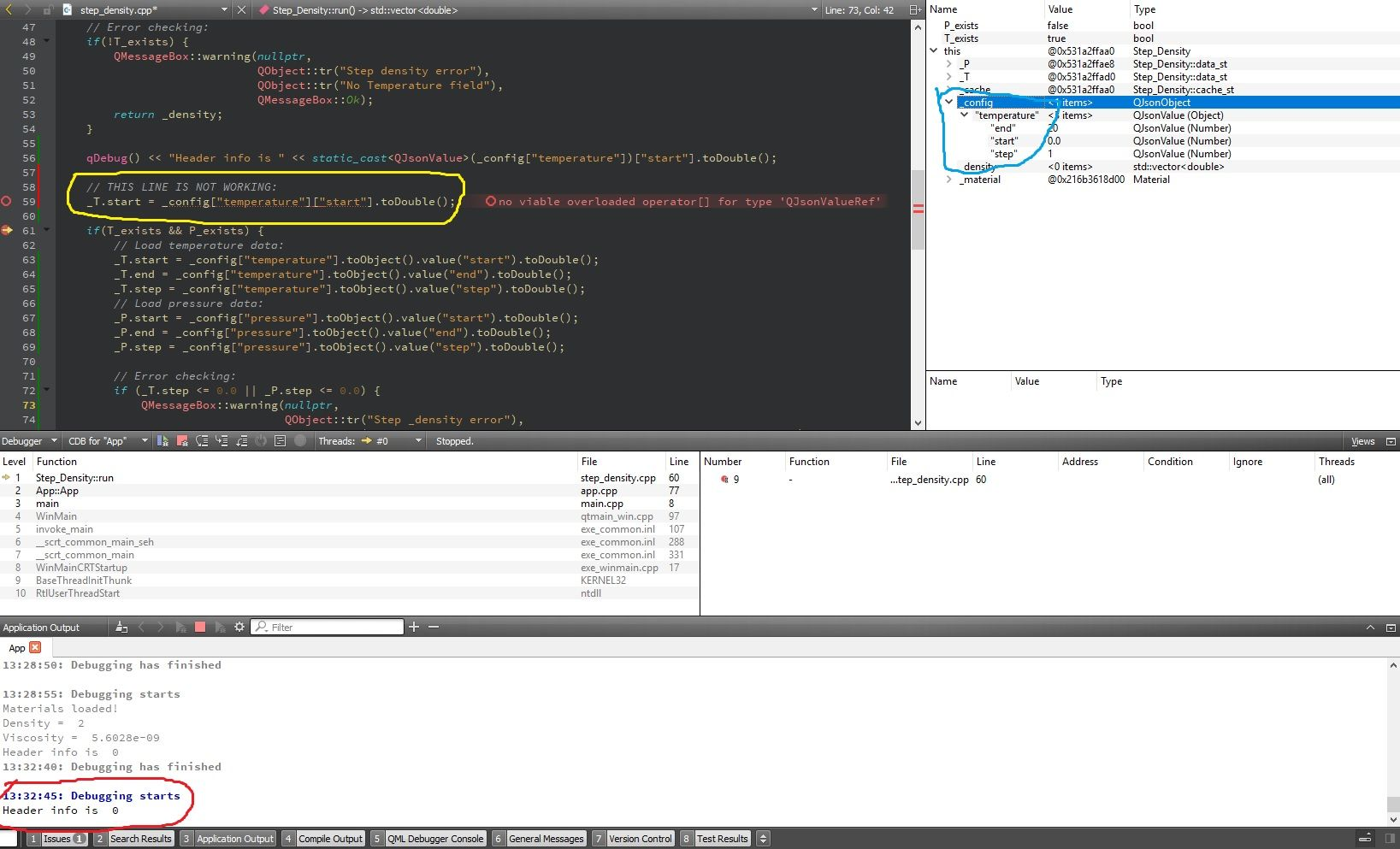# QJsonObject: Notation error

• Hi,

I'm using QJsonObject a lot, but now I got an notation error that is appearing only in one single Qt class of my project.

I'm always using this notation type:

``````double x = json["a"]["b"]["c"].toDouble();
``````

So, inside my Qt class I typed:

``````double start = config["temperature"]["start"].toDouble();
``````

But I got this error message:

``````error: no viable overloaded operator[] for type 'QJsonValueRef'
``````

If I change the JSON notation to this line below, it works good:

``````double start = config["temperature"].toObject().value("start").toDouble();
``````

Why this is happening? Why I can use the first notation above?

My class:

``````#include <QJsonObject>

class Step_Density
{
private:
QJsonObject _config;

public:
Step_Density();
};
``````

• Hi,

What does temperature contain ?

• @SGaist , the `config` is a `QJsonObject`.

The content of `config` is:

``````{
"temperature":
{
"start": 0.0,
"end": 100.0
}
}
``````

What is wrong in the first (clean) notation?

• This is curious, but I think the problem is that operator[] can return either a QJsonValue or a QJsonValueRef.
This seems to work:

``````    qDebug() << "Header info is " << static_cast<QJsonValue>(configObject["Header"])["Tag"].toString();
``````

So I think it's picking QJsonValueRef, since the documentation for QJsonValue clearly states that operator[] is
Equivalent to calling toObject().value(key).

• @mranger90 thank you,

I type:

``````qDebug() << "Header info is " << static_cast<QJsonValue>(_config["Header"])["temperature"].toString();
``````

and I got:

``````Header info is  ""
``````

Yes, the operator `[]` is Equivalent to calling `toObject().value(key)`, and I'm using this operator `[]` all the time in my project with `QJsonObject`.
My doubt is: Why in this `Step_Density` class the operator `[]` is returning a `QJsonValueRef` and not a `QJsonValue` as always was.

UPDATE 1:
Just to help to understand the error I remove the `start` node and I got no errors. Like:

``````double start = config["temperature"].toDouble();
``````

So, I think that the problem is related with the second JSON node...
What it ca be?

• This works for me (Qt 5.13.0, ubuntu 19.04)

``````
QJsonObject configObject = jsonDoc.object();
if (configObject.isEmpty())
{
qDebug() << "Json file is empty or root type is not an object (i.e. {})." << configObject;
}

``````

where the json file is:

``````{
"Tag": "Release 1.0",
"Temperature": 42.24
}
}
``````

• @mranger90, I understand your notation, but I got no point about why this is happening now?

I uncommment the "wrong line" after the Debug starts only to be more visible in this image.Could you help me to use the first notation?

PS.: the type of the private member class called `_T.start` is `double`

• The first notation does not work because, for reasons that are beyond my level of C++ expertise, the return of QJsonObject::operator[] is being interpreted as a QJsonValueRef, NOT a QJsonValue. Hence the cast I made.
The only other suggestion is to do it in 2 steps:

``````QJsonValue tempVal = _config["Temperature"];
_T.start = tempVal["start"].toDouble();
_T.end = tempVal["end"].toDouble();
``````

• he only other suggestion is to do it in 2 steps:

Yes, I think that you are right...and your code works good. Thank you.

Well..I can use it...no problems! I just want to understand why this is hapenning...
I will let this post opened, ok?